## Everything

I use the Open Source photo management Software Digikam (along with other tools such as Gimp and DarkTable).  I obviously need very little encouragement to combine my geeky hobbies, so I quickly tried to interrogate Digikam with R, which is easy, because Digikam keeps all it's image info in a SQLite database, which R has support for.

So this post shows how I did it, along with some of the output, such as the focal length of my images over time, looks like I need a telephoto lens !  (this script and my digikam db are in github here)

``````
ggplot(data = subset(ImgMerge, model == "NIKON D5000" & focalLength < 60), aes(x = as.POSIXct(creationDate),
y = focalLength)) + geom_point(alpha = 0.2)
``````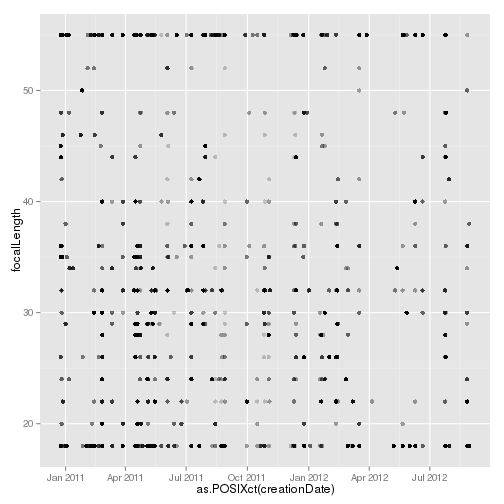``````
library(RSQLite)
``````
``````## Loading required package: DBI
``````

`library(ggplot2) library(plyr) m <- dbDriver("SQLite") basedir <- "/home/paul/RStudio/DigikamR/" con <- dbConnect(m, dbname = paste(basedir, "data/digikam4.db", sep = "")) `

Now we've opened the database, we can examine some of the tables within it.

``````
# List the tables in the database
dbListTables(con)
``````
``````##   "AlbumRoots"         "Albums"             "DownloadHistory"
##  "
ImagePositions"     "ImageProperties"    "ImageRelations"
##  "ImageTagProperties" "ImageTags"          "Images"
##  "Searches"           "Settings"           "TagProperties"
##  "Tags"               "TagsTree"
``````
``````
# List the columns of some of the interesting tables
``````
``````##   "imageid"          "rating"           "creationDate"
##   "digitizationDate" "orientation"      "width"
##   "height"           "format"           "colorDepth"
##  "colorModel"
``````
``````names(dbReadTable(con, "ImageComments"))
``````
``````##  "id"       "imageid"  "type"     "language" "author"   "date"
##  "comment"
``````
``````names(dbReadTable(con, "ImageMetadata"))
``````
``````##   "imageid"                      "make"
##   "model"                        "lens"
##   "aperture"                     "focalLength"
##   "focalLength35"                "exposureTime"
##   "exposureProgram"              "exposureMode"
##  "sensitivity"                  "flash"
##  "whiteBalance"                 "whiteBalanceColorTemperature"
##  "meteringMode"                 "subjectDistance"
##  "subjectDistanceCategory"
``````
``````names(dbReadTable(con, "ImageProperties"))
``````
``````##  "imageid"  "property" "value"
``````
``````names(dbReadTable(con, "ImagePositions"))
``````
``````##   "imageid"         "latitude"        "latitudeNumber"
##   "longitude"       "longitudeNumber" "altitude"
##   "orientation"     "tilt"            "roll"
##  "accuracy"        "description"
``````
``````names(dbReadTable(con, "Images"))
``````
``````##  "id"               "album"            "name"
##  "status"           "category"         "modificationDate"
##  "fileSize"         "uniqueHash"
``````
``````names(dbReadTable(con, "TagProperties"))
``````
``````##  "tagid"    "property" "value"
``````

And now we can pull some of the inetresting tables into a dataframe

``````
# Pull some of the information together
# and merge it together
ImgMerge <- merge(Imgs, ImgMeta, by.x = "id", by.y = "imageid")
ImgMerge <- merge(ImgMerge, ImgInfo, by.x = "id", by.y = "imageid")
# clean it up
ImgMerge\$make <- as.factor(ImgMerge\$make)
ImgMerge\$model <- as.factor(ImgMerge\$model)
ImgMerge\$faperture <- as.factor(ImgMerge\$aperture)
ImgMerge\$fexposureTime <- as.factor(ImgMerge\$exposureTime)
ImgMerge\$fmodel <- as.factor(ImgMerge\$model)
ImgMerge\$Year <- format(as.POSIXct(ImgMerge\$creationDate), format = "%Y")
ImgMerge\$Month <- format(as.POSIXct(ImgMerge\$creationDate), format = "%b")
``````

Here are some plots

``````# and draw some graphs
ggplot(data = subset(ImgMerge, focalLength < 60), aes(x = as.POSIXct(creationDate),
y = focalLength, colour = model)) + geom_point()
``````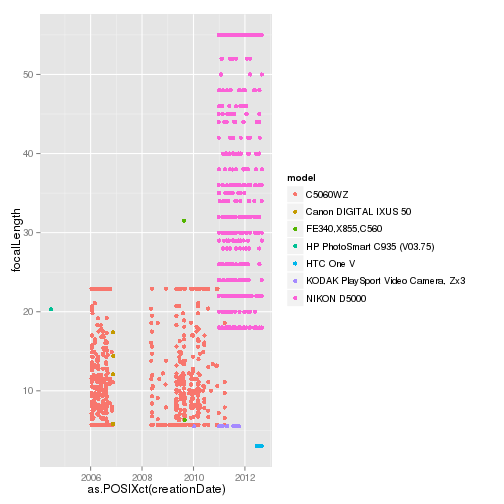``````
ggplot(data = ImgMerge, aes(x = focalLength)) + geom_histogram(binwidth = 5,
aes(colour = as.factor(model))) + facet_grid(model ~ .)
``````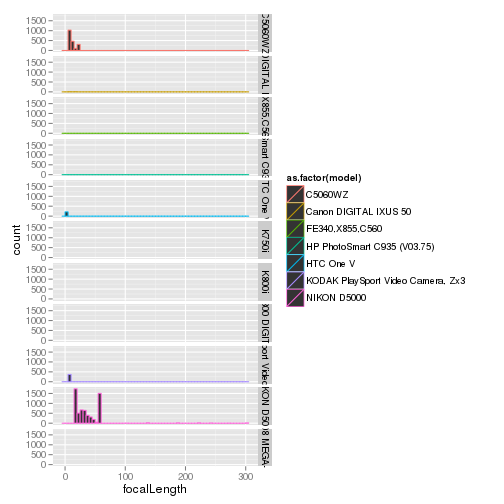``````
qplot(data = ImgMerge, x = as.numeric(as.character(aperture)), y = log(as.numeric(as.character(exposureTime))),
colour = as.factor(model), geom = "point")
``````
``````## Warning: Removed 2638 rows containing missing values (geom_point).
``````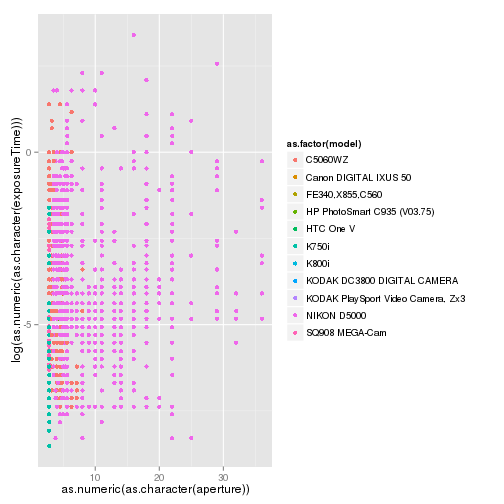``````
ggplot(data = subset(ImgMerge, model == "NIKON D5000"), aes(x = focalLength)) +
geom_histogram(binwidth = 5) + facet_grid(Year ~ .)
``````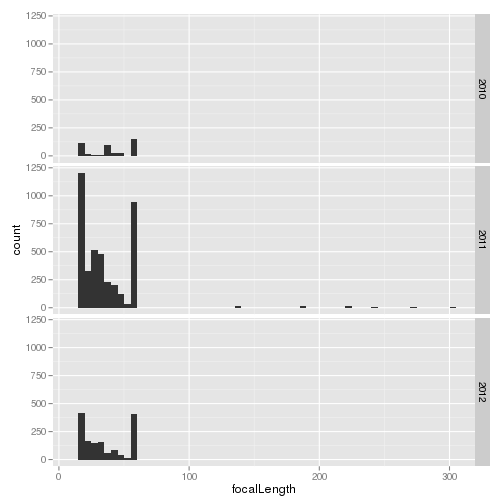``````
ggplot(data = subset(ImgMerge, model == "NIKON D5000"), aes(x = as.POSIXct(creationDate),
y = focalLength)) + geom_point()
``````
``````## Warning: Removed 14 rows containing missing values (geom_point).
``````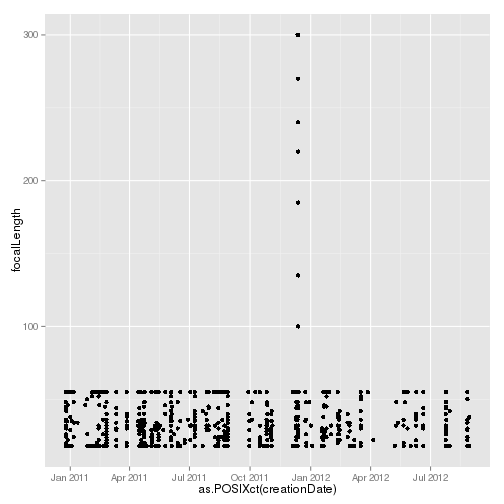``````
ggplot(data = subset(ImgMerge, model == "NIKON D5000" & focalLength < 60), aes(x = as.POSIXct(creationDate),
y = focalLength)) + geom_point(alpha = 0.2)
``````I recently wanted to create a ggplot that I could then 'tweak' furthur. This is my solution, to create an .svg file which can be loaded into a suitable application (I prefer Inkscape) and furthur edited / tweaked.

`# Build an example Plotlibrary(ggplot2)dataframe <- data.frame(fac = factor(c(1:4)), data1 = rnorm(400, 100, sd = 15))dataframe\$data2 <- dataframe\$data1 * c(0.25, 0.5, 0.75, 1)`
`testplot <- qplot(x = fac, y = data2, data = dataframe, colour = fac, geom = c("boxplot",     "jitter"))testplot``# Produce a PNG plotlibrary(Cairo)Cairo(800, 800, file = "testplot12200.png", type = "png", bg = "transparent",     pointsize = 12, units = "px", dpi = 200)testplotdev.off()``#Produce an svg filelibrary(Cairo)Cairo(800,800,file="cairo_2.svg",type="svg",bg="transparent",pointsize=12, units="in",dpi=400, width=20, height=20)testplotdev.off()`There are several ways to make a Stepper Motor run, and the best way will depend on the application, the motor and the electronics available. For running a stepper motor from an Arduino these are the main ways to go

1. A ULN2003 Darlington driver board. Typically sold with small geared steppers this requires four digital pins and the Arduino sketch needs to directly drive each coil

2. A driver board/shield with a constant voltage driver, such as the Adafruit Motor Shield. This runs over SPI (so only needs two pins) and can run many kinds of steppers and normal motors fine, unfortunately it couldn't run my steppers.

3. A 'chopper' driver that will vary the voltage to keep a constant current, such as the A4988 or the DRV8825 chip, either direct or via a board/shield such as the Stepstick or Pololu.

I wrote an instructable that covers the third method, running one or more steppers via an A4988 IC on a StepStick board.  See it here